# Please answer fully and clearly. Thank you. )if (e-) (0.0)) if (z, y)#10, 0), 2. Consider f R2R defined by f(,) plHP, 0, if (x,y) (0,0)J (a) Show by explicit computation that the directional derivati...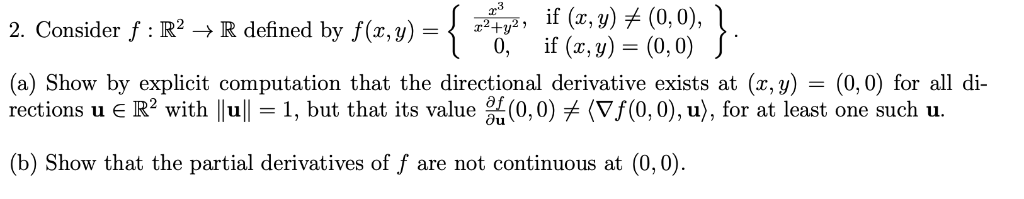)if (e-) (0.0)) if (z, y)#10, 0), 2. Consider f R2R defined by f(,) plHP, 0, if (x,y) (0,0)J (a) Show by explicit computation that the directional derivative exists at (x, y) - (0,0) for all di rections u R2 with lull-1, but that its value (0,0)メ(Vf(0,0), u), for at least one such u. b) Show that the partial derivatives of f are not continuous at (0,0)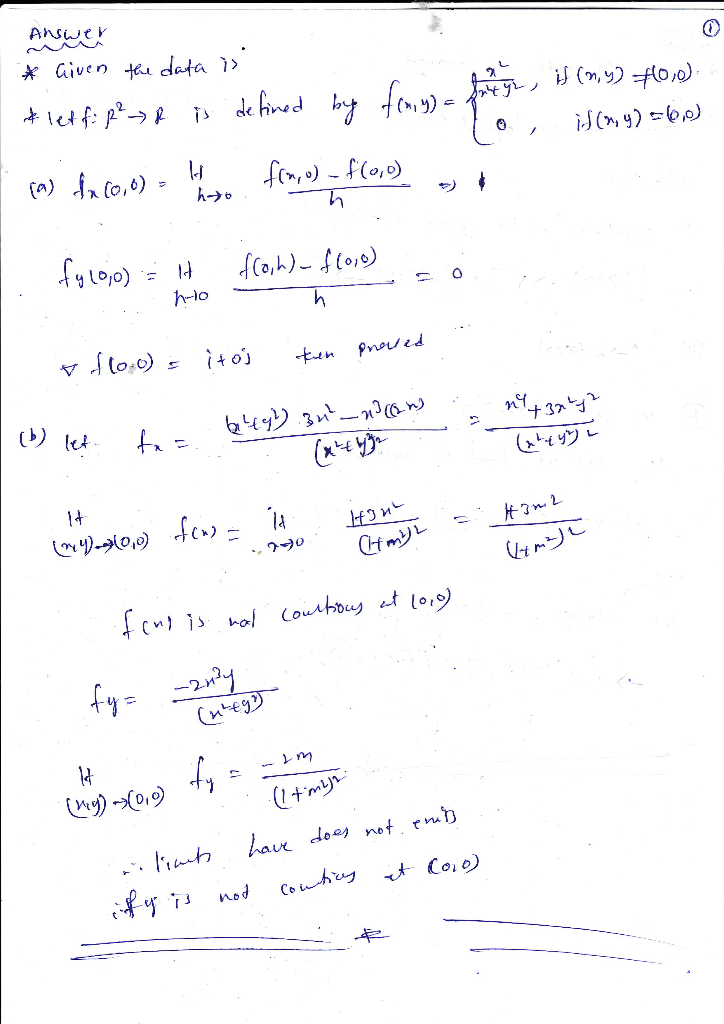#### Earn Coin

Coins can be redeemed for fabulous gifts.

Similar Homework Help Questions
• ### if (r.y) (0,0), 0,f (, y) (0, 0) 2. Consider f : IR2 -R defined by f(r,y)-+ (a) Show by explicit computation that the directional derivative exists at (x, y)- (0,0) for all oi rections u є R2 with 1...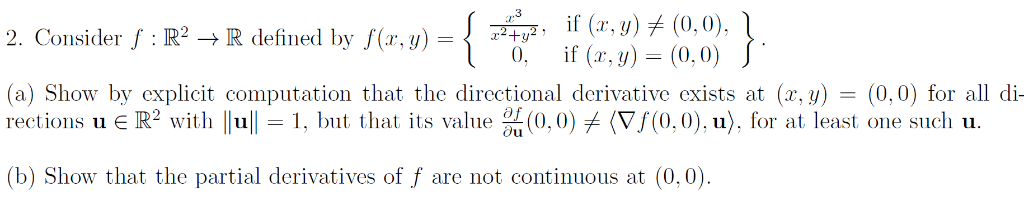if (r.y) (0,0), 0,f (, y) (0, 0) 2. Consider f : IR2 -R defined by f(r,y)-+ (a) Show by explicit computation that the directional derivative exists at (x, y)- (0,0) for all oi rections u є R2 with 1 11-1, but that its value %(0.0) (Vf(0,0).u), fr at least one sucli u. (b) Show that the partial derivatives of f are not continuous at (0,0) if (r.y) (0,0), 0,f (, y) (0, 0) 2. Consider f : IR2 -R...

• ### Consider the function f: RR given by if (x, y)メ(0,0) íf (z, y) = (0,0). f(z,...Consider the function f: RR given by if (x, y)メ(0,0) íf (z, y) = (0,0). f(z, y)=(0+r R2 does the directional derivative Duf(0,0) exist? Evaluate the For which vectors 0メu directional derivative wherever it exists. 4 marks

• ### Define f: R2R by 224V2 y) (0,0) 0 if (x, y)-(0,0) if (z, f(z, y) (a)...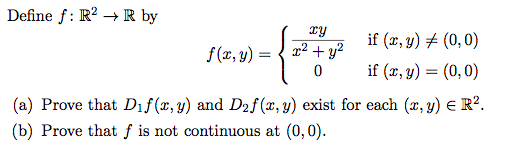Define f: R2R by 224V2 y) (0,0) 0 if (x, y)-(0,0) if (z, f(z, y) (a) Prove that Dif(z, y) and D2f (x, y) exist for each (x, y) E R2. (b) Prove that f is not continuous at (0,0).

• ### 2. Consider the function f : R2 → R defined below. r3уг_ if (x,y) (0,0) f(x,y)...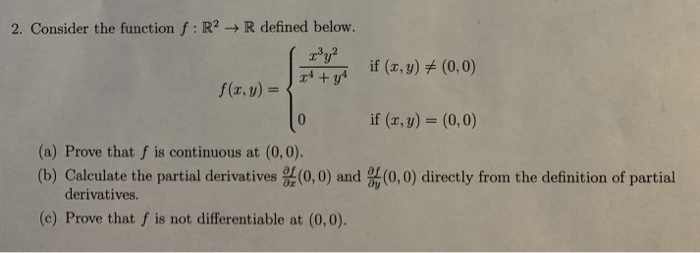2. Consider the function f : R2 → R defined below. r3уг_ if (x,y) (0,0) f(x,y) = if (x, y) (0, 0) (a) Prove that f is continuous at (0,0) (b) Calculate the partial derivatives (0,0) and (0,0) directly from the definition of partial derivatives. (c) Prove that f is not differentiable at (0,0).

• ### Exercice 2 (5pts) Let f given by f(x, y) Isinyif (x, y) (0,0) and f(0,0) 0...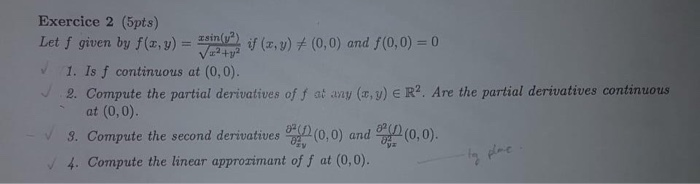Exercice 2 (5pts) Let f given by f(x, y) Isinyif (x, y) (0,0) and f(0,0) 0 1V224 1. Is f continuous at (0,0). 2. Compute the partial derivatives of f at any (x, y) E R2. Are the partial derivatives continuous (0,0). at (0,0) (0,0) and 3. Compute the second derivatives 4. Compute the linear approzimant of f at (0,0). Exercice 2 (5pts) Let f given by f(x, y) Isinyif (x, y) (0,0) and f(0,0) 0 1V224 1. Is f...

• ### Anyone can solve these questions? (4) Let if (z, y)メ(0,0) if (x, y) (0,0) f(z, y)...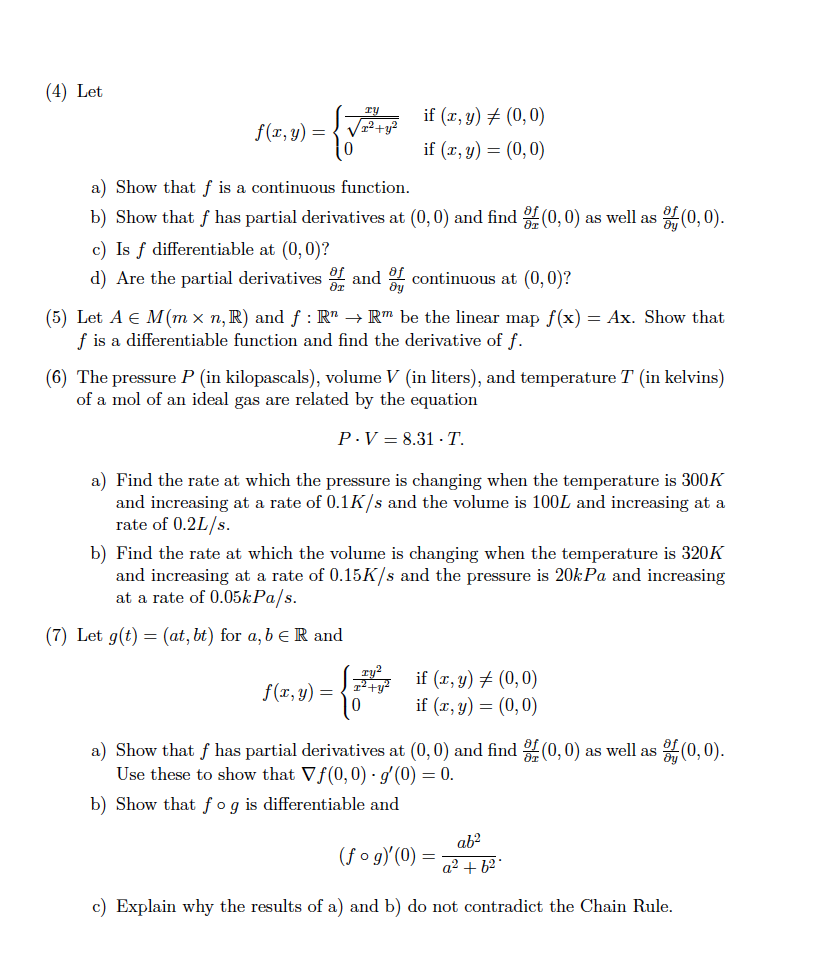Anyone can solve these questions? (4) Let if (z, y)メ(0,0) if (x, y) (0,0) f(z, y) / 0 a) Show that f is a continuous function b) Show that f has partial derivatives at (0,0) and find (0,0) as well as c) Is f differentiable at (0, 0)? d) Are the partial derivatives r tinuous at (0,0)? (0,0) (5) Let A E M(mx n,R) and f : RnRm be the linear map f(x)-Ax. Show that f is a differentiable function...

• ### b) i. Using e-8 definition show that f is continuous at (0,0), where f(x,y) = {aš...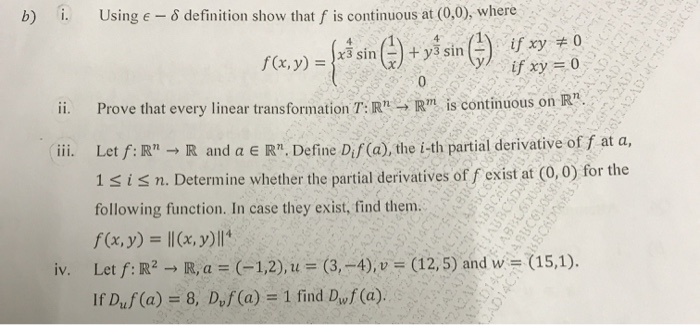b) i. Using e-8 definition show that f is continuous at (0,0), where f(x,y) = {aš sin () + yś sin () if xy + 0 242ADES if xy = 0 ii. Prove that every linear transformation T:R" - R" is continuous on R". iii. Let f:R" → R and a ER" Define Dis (a), the i-th partial derivative of f at a, 1 sisn. Determine whether the partial derivatives of f exist at (0,0) for the following function. In...

• ### DUE DATE: 23 MARCH 2020 1 1. Let f(x,y) = (x, y) + (0,0) 0. (x,...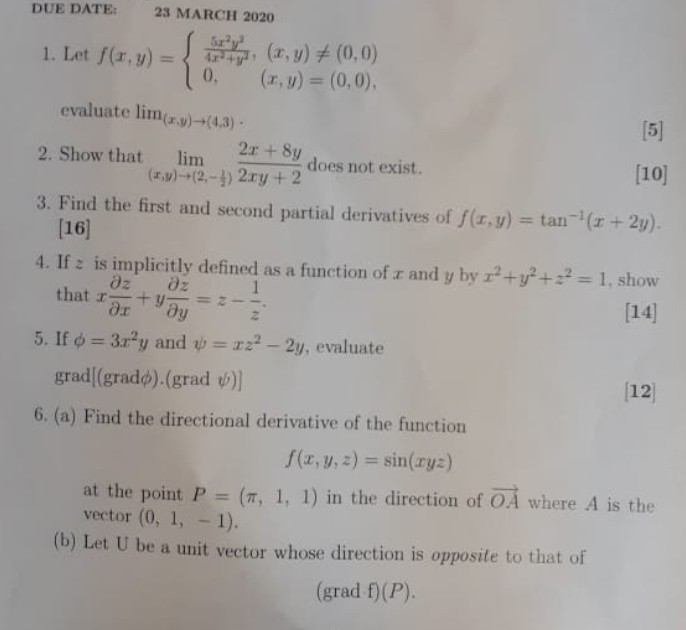DUE DATE: 23 MARCH 2020 1 1. Let f(x,y) = (x, y) + (0,0) 0. (x, y) = (0,0) evaluate lim(x,y)=(4,3)  2r + 8y 2. Show that lim does not exist.  (*.w)-(2,-1) 2.ry + 2 3. Find the first and second partial derivatives of f(x,y) = tan-'(x + 2y).  4. If z is implicitly defined as a function of x and y by I?+y2 + 2 = 1, show az Əz that +y=z  ar ду 5....

• ### (1 point) Consider the function defined by F(x, y) = x2 + y2 except at (r, y) - (0, 0) where F(0,0)0 Then we have (0,0)...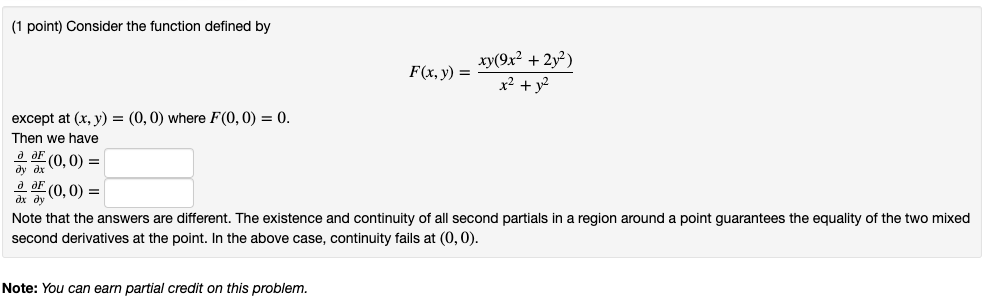(1 point) Consider the function defined by F(x, y) = x2 + y2 except at (r, y) - (0, 0) where F(0,0)0 Then we have (0,0) = (0,0) = ax dy Note that the answers are different. The existence and continuity of all second partials in a region around a point guarantees the equality of the two mixed second derivatives at the point. In the above case, continuity fails at (0,0) Note: You can earn partial credit on this problem...

• ### Question 2 (20 points): Consider the functions f(x, y)-xe y sin y and g(x, y)-ys 1. Show f is differentiable in its domain 2. Compute the partial derivatives of g at (0,0) 3. Show that g is not d...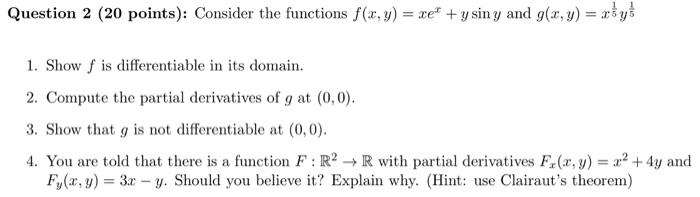Question 2 (20 points): Consider the functions f(x, y)-xe y sin y and g(x, y)-ys 1. Show f is differentiable in its domain 2. Compute the partial derivatives of g at (0,0) 3. Show that g is not differentiable at (0,0) 4. You are told that there is a function F : R2 → R with partial derivatives F(x,y) = x2 +4y and Fy(x, y 3x - y. Should you believe it? Explain why. (Hint: use Clairaut's theorem) Question 2...